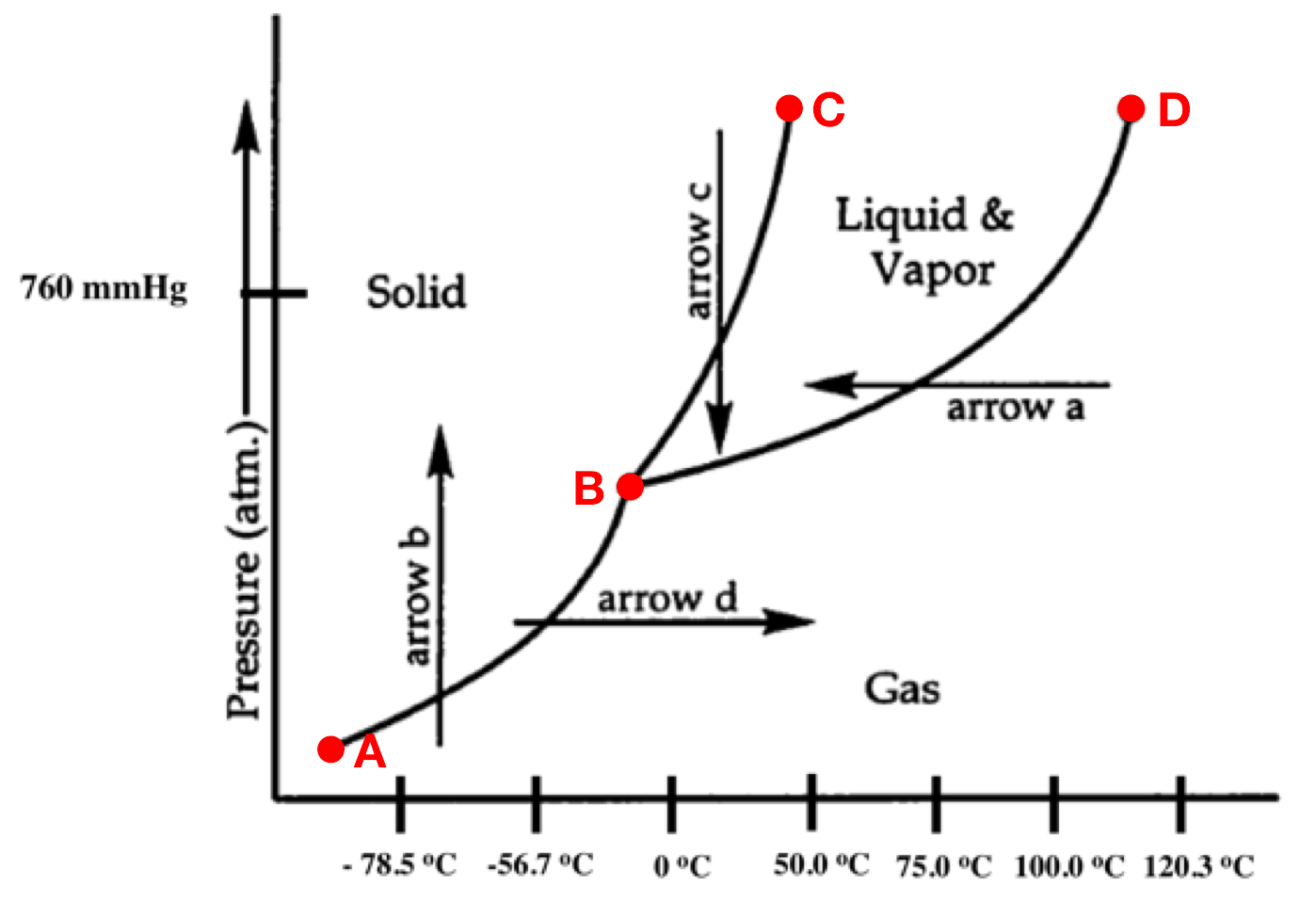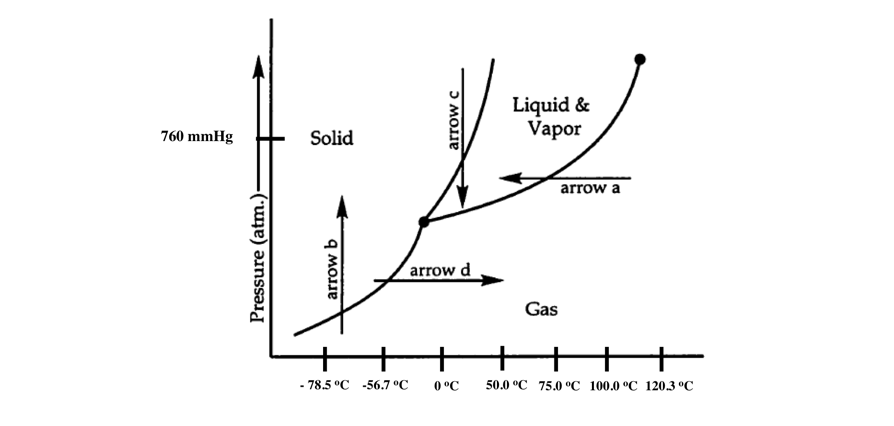# Problem: Answer the following questions based on the following phase diagram given below.a. At what temperature can we no longer tell the difference between the liquid and the gas? _____________b. Which point represents an equilibrium between the solid, liquid, and gas phase?    _____________c. Which line segment represents an equilibrium between fusion and freezing? _____________d. Which line segment represents an equilibrium between sublimation and deposition? _____________e. Which line segment represents an equilibrium between condensation and vaporization? _____________f. What is the normal freezing point of this unknown substance? _____________g. What is the normal boiling point of this unknown substance? _____________

###### FREE Expert Solution
80% (147 ratings)
###### FREE Expert Solution

We can use the phase diagram to answer the given questions

Recall that a phase diagram shows the transition of matter between solid, liquid, and gas phases as temperature and pressure changes.

In a phase diagram, the different phases can be identified by their location:

• Solid: can be found at high pressure and low temperature

• Liquid: can be found between the solid and gas regions

• Gas: can be found at low pressure and high temperature

The given phase diagram is:80% (147 ratings)###### Problem Details

Answer the following questions based on the following phase diagram given below.

a. At what temperature can we no longer tell the difference between the liquid and the gas? _____________

b. Which point represents an equilibrium between the solid, liquid, and gas phase?    _____________

c. Which line segment represents an equilibrium between fusion and freezing? _____________

d. Which line segment represents an equilibrium between sublimation and deposition? _____________

e. Which line segment represents an equilibrium between condensation and vaporization? _____________

f. What is the normal freezing point of this unknown substance? _____________

g. What is the normal boiling point of this unknown substance? _____________What scientific concept do you need to know in order to solve this problem?

Our tutors have indicated that to solve this problem you will need to apply the Phase Diagram concept. You can view video lessons to learn Phase Diagram. Or if you need more Phase Diagram practice, you can also practice Phase Diagram practice problems.

What is the difficulty of this problem?

Our tutors rated the difficulty ofAnswer the following questions based on the following phase ...as high difficulty.

How long does this problem take to solve?

Our expert Chemistry tutor, Dasha took 6 minutes and 10 seconds to solve this problem. You can follow their steps in the video explanation above.

What professor is this problem relevant for?

Based on our data, we think this problem is relevant for Professor Mcintire's class at UCI.